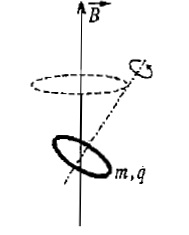# Larmor precessionIn homogenous magnetic field of induction $B$ thin charged ring is rotating around it's own axis. Mass of ring is $m$ and charge is $q$. Find the velocity of precession ring's axis around magnetic field line passing through the centar of ring.

$B=0.1T$

$q=3C$

$m=0.01kg$

×

Problem Loading...

Note Loading...

Set Loading...